# ICSE Solutions for Chapter 10 Electro Magnetism Class 10 Selina Physics

Question 1: Describe an experiment to demonstrate that there is a magnetic field around a current-carrying conductor.

Solution 1: Experiment:
In Fig, AB is a wire lying in the north-south direction and connected to a battery through a rheostat and a tapping key. A compass needle is placed just below the wire. It is observed that
(1) When the key is open i.e., no current passes through the wire, the needle shows no deflection and it points in the N-S direction (i.e. along the earth's magnetic field). In this position, the needle is parallel to the wire as shown in Fig. (a).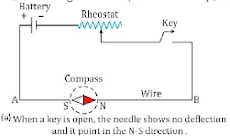(2) When the key is pressed, a current passes in the wire in the direction from A to B (i.e. From south to north) and the north pole(N) of the needle deflects towards the west [Fig. (b)].(3) When the direction of current in the wire is reversed by reversing the connections at the terminals of the battery, North Pole (N) of the needle deflects towards the east [Fig. (c)].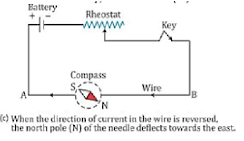(4) If the compass needle is placed just above the wire, the North Pole (N) deflects towards east when the direction of current in wire is from A to B [Fig. (d)], but the needle deflects towards west as in fig (e), if the direction of current in wire is from B to A.The above observations of the experiment suggest that a current-carrying wire produces a magnetic field around it.

Question 2: Draw a diagram showing the directions of three magnetic field lines due to a straight wire carrying current. Also show the direction of current in the wire.

Solution 2: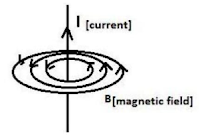Question 3: How is the magnetic field due to a straight current-carrying wire affected if current in wire is (a) decreased, (b) reversed?

Solution 3: (a) On decreasing the current the magnetic field lines become rarer. (b) The direction of magnetic field lines will get reversed.

Question 4: State a law, which determines the direction of magnetic field around a current-carrying wire.

Solution 4: Right hand thumb rule determines the direction of magnetic field around a current-carrying wire. It states that if we hold the current-carrying conductor in right hand such that the thumb points in the direction of flow of current, then the fingers encircle the wire in the direction of the magnetic fields lines.

Question 5: A straight wire lying in a horizontal plane carries a current from north to south. (a) What will be the direction of magnetic field at a point just underneath it? (b) Name the law used to arrive at the answer in part (a).

Solution 5: (a) The direction of magnetic field at a point just underneath is towards east.
(b) Right hand thumb rule.

Question 6: What will happen to a compass needle when the compass is placed below a wire and a current is made to flow through the wire? Give a reason to justify your answer.

Solution 6: A current carrying conductor produces a magnetic field around it and the magnetic needle in this magnetic field experience a torque due to which it deflects to align itself in the direction of magnetic field.

Question 7: Draw a labelled diagram showing the three magnetic field lines of a loop carrying current. Mark the direction of current and the direction of magnetic field by arrows in your diagram.

Solution 7:Question 8: A wire, bent into a circle, carries current in an anticlockwise direction. What polarity does this face of the coil exhibit?

Solution 8: Face of the coil exhibit North polarity.

Question 9: What is the direction of magnetic field at the centre of a coil carrying current in (i) clockwise, (ii) anticlockwise direction?

Solution 9:
(i) Along the axis of coil inwards.
(ii) Along the axis of coil outwards.

Question 10: Draw a diagram to represent the magnetic field lines along the axis of a current-carrying solenoid mark arrows to show the direction of current in the solenoid and the direction of magnetic field lines.

Solution 10: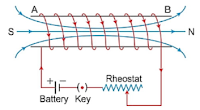Question 11: Name and state the rule by which polarity at the ends of a current carrying solenoid is determined.

Solution 11: Right hand thumb rule: If we hold the current carrying conductor in right hand such that the thumb points in the direction of flow of current, then the fingers encircle the wire in the direction of the magnetic fields lines.

Question 12: The adjacent diagram shows a small magnet placed near a solenoid AB. Current is switched on in the solenoid by pressing the key K. (a) State the polarity at the ends A and B. (b) will the magnet be attracted or repelled? Give a reason for your answer.

Solution 12: (a) A - North pole, B - South pole.
(b) The magnet will be repelled because the end of the solenoid near the north pole of magnet becomes the north pole as current at this face is anticlockwise and the two like poles repel.

Question 13: The following diagram shows a spiral coil wound on a hollow carboard tube AB. A magnetic compass is placed close to it. Current it switched on by closing the key. (a) What will be the polarity at the ends A and B? (b) How will the compass needle be affected? Give reason.Solution 13: (a) A - North pole, B - South pole.
(b) The north pole of compass needle will deflect towards west.
Reason: The end A of the coil behaves like north pole which repels north pole of compass needle towards west.

Question 14: State two ways by which the magnetic field due to a solenoid can be made stronger.

Solution 14: The magnetic field due to a solenoid can be made stronger by using: (i) By increasing the number of turns of winding in the solenoid. (ii) By increasing the current through the solenoid.

Question 15: Why does a current-carrying freely suspended solenoid rest along a particular direction?

Solution 15: A current-carrying freely suspended solenoid at rest behaves like a bar magnet. It is because a current-carrying solenoid behaves like a bar magnet.

Question 16: What effect will there be on magnetic compass when it is brought near a current carrying solenoid?

Solution 16: The needle of the compass will rest in in the direction of magnetic field due to solenoid at that point.

Question 17: How is the magnetic field due to a solenoid carrying current affected if a soft iron bar is introduced inside the solenoid?

Solution 17: Magnetic field due to a solenoid carrying current increases if a soft iron bar is introduced inside the solenoid.

Question 18: Complete the following sentences: (a) when current flows in a wire, it creates ........ (b) A current-carrying solenoid behaves like a ......... (c) A current-carrying solenoid when freely suspended, it always rests in .......... Direction.

Solution 18: (a) When current flows in a wire, it creates magnetic field.
(b) A current-carrying solenoid behaves like a bar magnet
(c) A current-carrying solenoid when freely suspended, it always rest in north-south direction.

Question 19: You are required to make an electromagnet from a soft iron bar by using a cell, an insulated coil of copper wire and a switch. (a) Draw a circuit diagram to represent the process. (b) label the poles of the electromagnet.

Solution 19:Question 20: The adjacent diagram shows a coil would around a soft iron bar XY. (a) State the polarity at the end X and Y as the switch is pressed. (b) Suggest one way increasing the strength of electromagnet so formed.Solution 20: (a) X-north pole, Y-south pole.
(b) By reducing resistance of circuit by mean of rheostat to increase current.

Question 21: (a) What name is given to a cylindrical coil of diameter less than its length? (b) If a piece of soft iron is placed inside the coil mentioned in part (a) and current is passed in the coil from a battery, what name is then given to the device so obtained? (c) Give one use of the device mentioned in part (b).

Solution 21: (a) Solenoid is a cylindrical coil of diameter less than its length. (b) The device so obtained is electromagnet. (c) It is used in electric relay.

Question 22: Show with the aid of a diagram how a wire is wound on a U-shaped piece of soft iron in order to make it an electromagnet. Complete the circuit diagram and label the poles of the electromagnet.

Solution 22: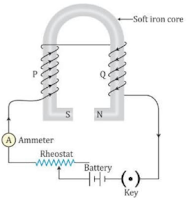Question 23: What is an electromagnet? Name two factors on which the strength of magnetic field of an electromagnet depends and state how does it depend on the factors stated by you.

Solution 23: An electromagnet is a temporary strong magnet made from a piece of soft iron when current flows in the coil wound around it. It is an artificial magnet. The strength of magnetic field of an electromagnet depends on:
(i) Number of turns: The strength of magnetic increases on increasing the number of turns of winding in the solenoid.
(ii) Current: The strength of magnetic field increases on increasing the current through the solenoid.

Question 24: Fig. shows the current flowing in the coil of wire wound around the soft iron horse shoe core. State the polarities developed at the ends A and B.Solution 24: At A-south pole and at B-north pole.

Question 25: State two ways through which the strength of an electromagnet can be increased.

Solution 25: the strength of an electromagnet can be increased by following ways:
(i) By increasing the number of turns of winding in the solenoid.
(ii) By increasing the current through the solenoid.

Question 26: State three uses of an electromagnet?

Solution 26:
Electromagnets are used in all kinds of electric devices, including hard disk drives, speakers, motors, and generators, as well as in scrap yards to pick up heavy scrap metal. They're even used in MRI machines, which utilize magnets to take photos of your insides.

Question 27: State two advantages of an electromagnet over a permanent magnet.

Solution 27: 1. An electromagnet can produce a strong magnetic field. 2. The strength of the magnetic field of an electromagnet can easily be changed by changing the current in its solenoid.

Question 28: State two differences between an electromagnet and a permanent magnet.

Solution 28:
 Electromagnet Permanent magnet It is made up of soft iron. It is made up of steel. The magnetic field strength can be changed. The magnetic field strength cannot be changed. The electromagnets of very strong field can be made. The permanent magnets are not so strong.

Question 29: Why is soft iron used as the core of the electromagnet in an electric bell?

Solution 29: The soft iron bar acquires the magnetic properties only when an electric current flows through the solenoid and loses the magnetic properties as the current is switched off. That's why soft iron is used as the core of the electromagnet in an electric bell.

Question 30: How is the working of an electric bell affected, if alternating current be used instead of direct current?

Solution 30: If an a.c. source is used in place of battery, the core of electromagnet will get magnetized, but the polarity at its ends will change. Since attraction of armature does not depend on the polarity of electromagnet, so the bell will still ring on pressing the switch.

Question 31: The incomplete diagram of an electric bell is given in Fig. Complete the diagram and label its different parts.Solution 31:Question 32: Name the material used for making the armature of an electric bell. Give a reason for your answer.

Solution 32: The material used for making the armature of an electric bell is soft iron which can induce magnetism rapidly.

Multiple Choice Type

Question 1: The present of magnetic field at a point can be detected by: (a) a strong magnet (b) a solenoid (c) a compass needle (d) a current-carrying wire

Solution 1: The presence of magnetic field at a point can be detected by a compass needle.
Note: In the presence of a magnetic field, the needle of compass rests only in the direction of magnetic field and in the absence of any magnetic field, the needle of compass can rest in any direction. In the earth's magnetic field alone, the needle rests along north-south direction.

Question 2: By reversing the direction of current in a wire, the magnetic field produced by it:
(a) gets reversed in direction
(b) increases in strength
(c) decreases in strength
(d) remains unchanged in strength and direction

Solution 2: By reversing the direction of current in a wire, the magnetic field produced by it gets reversed in direction.
Hint: On reversing the direction of current in a wire, the polarity of the faces of the wire also reverses. Thus, the direction of magnetic field produced by it also gets reversed.

### Exercise 10 B

Question 1: Name three factors on which the magnitude of force on a current-carrying conductor placed in a magnetic field depends and state how does the force depend on the factors stated by you.

Solution 1: The magnitude of force on a current-carrying conductor placed in a magnetic field depends on:
(i) On strength of magnetic field B.
(ii) On current I in the conductor.
(iii) On length of conductor.
Magnitude of force on a current-carrying conductor placed in a magnetic field depends directly on these three factors.

Question 2: State condition when magnitude of force on a current-carrying conductor placed in a magnetic field is (a) Zero (b) maximum.

Solution 2: (a) When current in the conductor is in the direction of magnetic field force will be zero.
(b) When current in the conductor is normal to the magnetic field.

Question 3: Name and state the law which is used to determine the direction of force on a current-carrying conductor placed in a magnetic field.

Solution 3: Fleming's left hand rule: Stretch the forefinger, middle finger and the thumb of your left hand mutually perpendicular to each other. If the forefinger indicates the direction of magnetic field and the middle finger indicates the direction of current, then the thumb will indicate the direction of motion of conductor.

Question 4: How will the direction of force be changed, if the current is reversed in the conductor placed in a magnetic field?

Solution 4: Direction of force is also reversed.

Question 5: State the unit of magnetic field in terms of the force experienced by a current-carrying conductor placed in a magnetic field

Solution 5: Unit is: Newton/ampere x meter (or NA-1m-1).

Question 6: A flat coil ABCD is freely suspended between the pole pieces of U-Shaped permanent magnet with the plane of coil parallel to the magnetic field. (a) What happens when a current is passed in the coil? (b) when will the coil come to rest? (c) when will the couple acting on the coil be (i) maximum (ii) minimum? (d) Name an instrument which makes use of the principle stated above.

Solution 6: (a) The coil will experience a torque due to which it will rotate.
(b) The coil will come to rest when their plane become normal to the magnetic field.
(c) (i) When plane of a oil is parallel to the magnetic field,
(ii) When plane of coil is normal to the magnetic field.
(d) The instrument which makes use of the principle stated above is d.c. motor.

Question 7: A coil ABCD mounted on an axle is placed between the poles N and S of a permanent magnet as shown in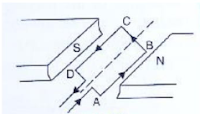(a) In which direction will the coil begin to rotate when current is passed through the coil in direction ABCD by connecting a battery at the ends A and D of the coil?
(b) Why is a commutator necessary for continuous rotation of the coil?
(c) Complete the diagram with commutator, ets. For the flow of current in the coil?

Solution 7: (a)The coil begins to rotate in anticlockwise direction.
(b) This is because, after half rotation, the arms AB and CD get interchanged, so the direction of torque on coil reverses. To keep the coil rotating in same direction, commutator is needed to change the direction of current in the coil after each half rotation of coil.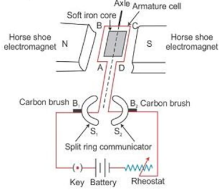Question 8: What is an electric motor? State its principle.

Solution 8: Electric motor: An electric motor is a device which converts the electrical energy into the mechanical energy.
Principle: An electric motor (dc motor) works on the principle that when an electric current is passed through a conductor placed normally in a magnetic field, a force acts on the conductor as a result of which the conductor begins to move and mechanical energy is obtained.

Question 9: Draw a labelled diagram of a d.c. motor.

Solution 9: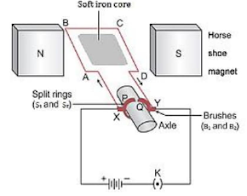Question 10: What energy conversion does take place during the working of a d.c. motor?

Solution 10: Electrical energy converts into mechanical energy.

Question 11: State two ways by which the speed of rotation of an electric motor can be increased?

Solution 11: The speed of rotation of an electric motor can be increased by:
(i) Increasing the strength of current.
(ii) Increasing the number of turns in the coil.

Question 12: Name two appliances in which an electric motor is used.

Solution 12: Electric motor is used in electrical gadgets like fan, washing machine, juicer, mixer, grinder etc.

Question 13: Complete the following sentence; ......... energy is converted into ............ energy by an electric motor.

Solution 13: Electrical energy is converted into mechanical energy by an electric motor.

Multiple Choice Type

Question 1: In an electric motor the energy transformation is:
(a) from electrical to chemical
(b) from chemical to light
(c) from mechanical to electrical
(d) from electrical to mechanical

Solution 1: In an electric motor, the energy transformation is from electrical to mechanical.
Note: An electric motor is a device which converts electrical energy into mechanical energy.

### Exercise 10 C

Question 1: (a) What is electromagnetic induction?
(b) Describe one experiment to demonstrate the phenomenon of electromagnetic induction.

Solution 1: (a) Electromagnetic induction: whenever there is change in number of magnetic field lines associated with conductor, an electromotive force is developed between the ends of the conductor which lasts as long as the change is taking place.
(b) Demonstration of the phenomenon of electromagnetic induction:
In the figure: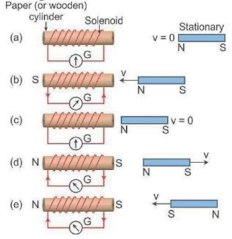(i) When the magnet is stationary there is no deflection in galvanometer. The pointer read zero. [Fig. (a)]
(ii) when the magnet with north pole facing the solenoid is moved towards the solenoid, the galvanometer shows a deflection towards the right showing that a current flows in the solenoid in the direction as shown in [Fig (b)]
(iii) As the motion of magnet stops, the pointer of the galvanometer comes to the zero position
[Fig (c)]. This shows that the current in the solenoid flows as long as the magnet is moving.
(iv) If the magnet is moved away from the solenoid, the current again flows in the solenoid, but
now in a direction opposite to that shown in [Fig. (b)] and therefore the pointer of the
galvanometer deflects towards left[ Fig. (d)].
(v) If the magnet is moved away rapidly i.e. with more velocity, the extent of deflection in the
galvanometer increases although the direction of deflection remains the same. It shows that more current flows now.
(vi) If the polarity of the magnet is reversed and then the magnet is brought towards the solenoid, the current in solenoid flows in the direction opposite to that shown in Fig (b) and so the pointer of galvanometer deflect towards left [Fig. (e)].

Question 2: State Faraday's law of electromagnetic induction.

Solution 2: Faraday's formulated two laws of electromagnetic induction:
(i) Whenever there is a change in the magnetic flux linked with a coil, an e.m.f. is induced. The induced e.m.f. lasts so long as there is a change in the magnetic flux linked with the coil.
(ii) The magnitude of the e.m.f. induced is directly proportional to the rate of change of the magnetic flux linked with the coil. If the rate of change of magnetic flux remains uniform, a steady e.m.f. is induced.

Question 3: State two factors on which the magnitude of induced e.m.f. depend.

Solution 3: Magnitude of induced e.m.f depend upon:
(i) The change in the magnetic flux.
(ii) The time in which the magnetic flux changes.

Question 4: (a) What kind of energy change takes place when a magnet is moved towards a coil having a galvanometer at its ends?
(b) Name the phenomenon

Solution 4: (a) Mechanical energy changes to the electrical energy.
(b) Phenomenon is called electromagnetic induction.

Question 5: (a) How would you demonstrate that a momentary current can be obtained by the suitable use of a magnet, a coil of wire and a galvanometer?
(b) What is the source of energy associated with the current obtained in part(a)?

Solution 5: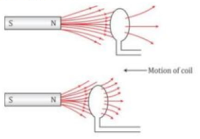(a) When there is a relative motion between the coil and the magnet, the magnetic flux linked with the coil changes. If the north pole of the magnet is moved towards the coil, the magnetic flux through the coil increases as shown in above figure. Due to change in the magnetic flux linked with the coil, an e.m.f. is induced in the coil. This e.m.f. causes a current to flow in the coil if the circuit of the coil is closed.
(b)The source of energy associated with the current obtained in part (a) is mechanical energy.

Question 6: (a) Describe briefly one way producing an induced e.m.f
(b) State one factor that determines the magnitude of induced e.m.f
(c) What factor determines the direction of induced e.m.f?

Solution 6: (a) Induced current can be produced by following activity: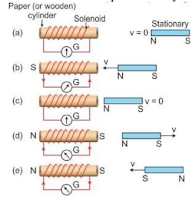(i) When the magnet is stationary there is no deflection in galvanometer. The pointer read zero. [Fig (a)]
(ii) When the magnet with north pole facing the solenoid is moved towards the solenoid, the
galvanometer shows a deflection towards the right showing that a current flows in the
solenoid in the direction as shown in Fig (b)
(iii) As the motion of magnet stops, the pointer of the galvanometer to the zero position [Fig(c)]. This shows that the current in the solenoid flows as long as the magnet is moving.
(iv) If the magnet is moved away from the solenoid, the current again flows in the solenoid, but now in the direction opposite to that shown in [Fig. (b)] and therefore the pointer of the galvanometer deflects towards left [Fig. (d)].
(v) If the magnet is moved away rapidly i.e. with more velocity, the extent of deflection in
the galvanometer increases although the direction of deflection remains the same. It
shows that more current flows now.
(vi) If the polarity of the magnet is reversed and then the magnet is brought towards the
solenoid, the current in solenoid flows in the direction opposite to that shown in Fig (b)
and so the pointer of galvanometer deflect towards left [Fig. (e)].
(b) Magnitude of induced e.m.f depend upon:
(i) The change in the magnetic flux.
(ii)The time in which the magnetic flux changes.
(c) The direction of induced e.m.f depends on whether there is an increase or decrease in the magnetic flux.

Question 7: Complete the following sentence: The current induced in a closed circuit only if there is ..........

Solution 7: The current induced in a closed circuit only if there is change in number of magnetic field lines linked with the circuit.

Question 8: In which of the following cases does the electromagnetic induction occur? (i) A current is started in a wire held near a loop of wire (ii) The current is stopped in a wire held near a loop of wire (iii) A magnet is moved through a loop of wire (iv) A loop of wire is held near a magnet.

Solution 8: (i) Yes.
(ii) Yes.
(iii) Yes.
(iv) No.

Question 9: A conductor is moved in a varying magnetic field. Name the law which determines the direction of current induced in the conductor.

Solution 9: Fleming's right hand rule determines the direction of current induced in the conductor.

Question 10: State Fleming's right hand rule.

Solution 10: Fleming's right hand rule: Stretch the forefinger, middle finger and the thumb of your right hand mutually perpendicular to each other. If the forefinger indicates the direction of magnetic field and the thumb will indicates the direction of motion of conductor, then the middle finger indicates the direction of induced current.

Question 11: What is Lenz's law?

Solution 11: Lenz's law: It states that the direction of induced e.m.f. (or induced current) is such that it always tends to oppose the cause which produces it.

Question 12: Why is it more difficult to move a magnet towards a coil which has large number of turns?

Solution 12: When a coil has a large number of turns, then magnitude of induced e.m.f. in the coil become more and then by Lenz's law it will oppose more.

Question 13: Explain why an induced current must flow in such a direction so as to oppose the change producing it.

Solution 13: So that the mechanical energy spent in producing the change, is transformed into the electrical energy in form of induced current.

Question 14: Explain the significance of Lenz's law to show the conservation of energy in electromagnetic induction.

Solution 14: Lenz's law implies the law of conservation of energy. It shows that the mechanical energy spent in doing work, against the opposing force experienced by the moving magnet, is transformed into the electrical energy due to which current flows in the solenoid.

Question 15: The following diagram in Fig.10.42 shows a coil of several turns of copper wire connected to a sensitive centre zero galvanometer G near a magnet NS. The coil is free to move in the direction shown in the diagram.(i) Describe the observation if the coil is rapidly moved.
(ii) How would the observation be altered if (a) the coil has twice as many turns (b the coil was made to move three times as fast?

Solution 15: (i) The pointer of galvanometer deflects. The deflection last so long as the coil moves.
(ii) (a) Deflection becomes twice.
(b) Deflection becomes thrice

Question 16: The following diagram in Fig. shows a fixed coil of several turns connected to a centre zero galvanometer G and a magnet NS which can move in the direction shown in the diagram.
(a) Describe the observation in the galvanometer if (i) the magnet is moved rapidly,(ii) the magent is kept still after it has moved into the coil, (iii) the magent is then rapidly pulled out of the coil.(b) How would the observation in (i) of part (a) alter if a more powerful magnet is used?

Solution 16: (i)The pointer of galvanometer deflects towards left. The deflection lasts so long as the coil moves.
(ii) (a) Deflection becomes twice (b) Deflection becomes thrice.

Question 17: The following diagram in Fig. shows a coil X connected to a sensitive centre –zero galvanometer G and a coil P connected to a battery through a switch S.(a) Describe the observation when the switch S is (i) closed suddenly, (ii) then kept closed, (iii) finally opened.
(b) Name and state the law which explains the above observations.

Solution 17: (a)
(i) When switch is closed suddenly, the galvanometer needle deflects for a moment.
(ii) If switch is kept closed then galvanometer needle returns to zero.
(iii) If switch is opened again then galvanometer needle deflects again but in opposite to the direction of deflection in case (a).
(b) This can be explained by Faraday's law which states that whenever there is change in the magnetic flux linked with a coil, an e.m.f. is induced. The induced e.m.f. lasts as long as there is a change in the magnetic flux linked with the coil.

Question 18: Name and state the principle of a simple a.c. generator what is its use?

Solution 18: An A.C. generator works on the principle of 'electromagnetic induction'.
Statement: Whenever a coil is rotated in a magnetic field, the magnetic flux linked with the coil changes, and therefore, an EMF is induced between the ends of the coil. Thus, a generator acts like a source of current if an external circuit containing load is connected between the ends of its coil.

Question 19: What determines the frequency of a.c. produced in a generator?

Solution 19: The number of rotations of the coil in one second or the speed of rotation of the coil.

Question 20: Draw a labelled diagram of a simple a.c. generator.

Solution 20:Question 21: In an a.c. generator the speed at which the coil rotates is doubled. How would this affect (a) the frequency of output voltage, (b) the maximum output voltage is doubled.

Solution 21: (a)In an a.c generator, if the speed at which the coil rotates is doubled, the frequency is also doubled.
(b) Maximum output voltage is also doubled.

Question 22: Suggest two ways in an a.c. generator to produce a higher e.m.f.

Solution 22: Two ways in an a.c generator to produce a higher e.m.f. are:
(1) By increasing the speed of rotation of the coil.
(2) By increasing the number of turns of coil.

Question 23: What energy conversion does take place in a generator when it is in use?

Solution 23: Mechanical energy changes into the electrical energy.

Question 24: State two dis-similarities and one similarity between a d.c. motor and an a.c. generator.

Solution 24: Two dissimilarities between D.C. motor and A.C. generator:
 AC Generator DC Motor A generator is a device which converts mechanical energy into electrical energy. A DC Motor is a device which converts electrical energy into mechanical energy. A generator works on the principle of electromagnetic induction. A DC motor works on the principle of force acting on a current carrying conductor placed in a magnetic field.

Question 25: State one advantage of using a.c. over d.c.

Solution 25: The voltage of a.c. can be stepped up by the use of step-up transformer at the power generating station before transmitting it over long distances. It reduces the loss of electrical energy as heat in the transmission line wires. On the other hand, if d.c. is generated at the power generating station, its voltage cannot be increased for transmission, and so due to passage of high current in the transmission line wires, there will be a huge loss of electrical energy as heat in the line wires.

Question 26: For what purpose are the transformers used? Can they be used with a direct current source?

Solution 26: The purpose of the transformer is to step up or step down the a.c. voltage. No, a transformer cannot be used with a direct current source.

Question 27: How are the e.m.f in the primary and secondary coils of a transformer related with the number of turns in these coils?

Solution 27: The relation is following:
ES/EP = NS/NP
S stands for secondary and P stands for primary.

Question 28: Draw a labelled diagram to show the various components of a step-up transformer

Solution 28: Step up transformer:Question 29: Name the device used to transform 12 V a.c. to 200V a.c. Name the principal on which it works.

Solution 29: The device is step up transformer. It works on the principle of electromagnetic induction.

Question 30: Draw a labelled diagram of a step-up transformer and explain how it works. State two characteristics of the primary coil as compared to its secondary coil.

Solution 30:Step-up transformer: The step-up transformer is used to change a low voltage alternating e.m.f. to a high voltage alternating e.m.f. of same frequency.
Working: When the terminals of primary coil are connected to the source of alternating e.m.f., a varying current flows through it which also produces a varying magnetic field in the core of the transformer. Thus, the magnetic field lines linked with the secondary coil vary and induce an e.m.f. in the secondary coil. The induced e.m.f. varies in the same manner as the applied e.m.f. in the primary coil varies, and thus, has the same frequency as that of the applied e.m.f. The magnitude of e.m.f. induced in the secondary coil depends on the 'turns ratio' and the magnitude of the applied e.m.f.
For a transformer,
Emf. across the secondary coil (ES)/Emf.across the primary coil(EP) =  Number of turns in the secondary coil (NS)/Number of turns in the primary coil (Np) = Turns ratio (n)
Two characteristics of the primary coil as compared to its secondary coil:
1. The number of turns in the primary coil is less than the number of turns in the secondary coil. 2. thicker wire is used in the primary coil as compared to that in the secondary coil.

Question 31: Draw a labelled diagram of a device you would use to transform 200V a.c. to 15V a.c. Name the device and explain how it works. Give its tow uses.

Solution 31: The device is step down transformer.Working: In a stepdown transformer, the number of turns in secondary coil are less than the number of turns in the primary coil i.e., turns ratio n<l or NS/NP<1.
As ES/EP = NS/NP.
So ES/Ep<1 which means ES is less than EP.
Two uses of step down transformer are:
(i) With electric bells
(ii) At the power sub-stations to step-down the voltage before its distribution to the customers.

Question 32: Complete the following diagram in Fig. of a transformer and name the parts labelled A and B. name the part you have drawn to complete the diagram. What is the material of this part? Is this transformer a step up or step down? Give reason.Solution 32: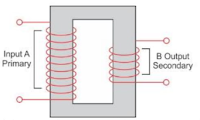A is the primary coil. B is the secondary coil.
We have drawn laminated core in the diagram.
The material of this part is soft iron.
This transformer a step-down transformer because the number of turns in primary coil is much greater than that in the secondary coil.

Question 33: The diagram below in Fig. shows the core of a transformer and its input and output connections.(a) state the material used for core and describe its structure.
(b) complete the diagram of the transformer and connections by labelling all parts joined by you.
(c) Name the transformer: Step-up or step down?

Solution 33: (a) Soft iron core is used. The core is made up from the thin laminated sheets of soft iron of T and U shape, placed alternately one above the other and insulated from each other by paint or varnish coating over them.
(b)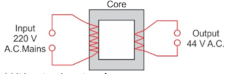(c) It is a step down transformer.

Question 34: The secondary windings of a transformer in which the voltage is stepped down are usually made of thicker wire than the primary. Explain why.

Solution 34: The secondary windings of a transformer in which the voltage is stepped down are usually made up of thicker than the primary because more current flows in the secondary coil. The use of thicker wire reduces its resistance and therefore the loss of energy as heat in the coil.

Question 35: Why is the iron core of a transformer made laminated (thin sheets) instead of being in one solid piece?

Solution 35: To reduce the energy losses due to eddy currents.

Question 36: Complete the following sentences:
(i) In a step-up transformer, the number of turns in the primary are ............than the number of turns in the secondary.
(ii) The transformer is used in ................. current circuits.
(iii) ……...... energy is converting into ............. energy by an electric generator.

Solution 36:
(i) In a step-up transformer, the number of turns in the primary is less than the number of turns in the secondary.
(ii) The transformer is used in alternating current circuits.
(iii) Mechanical energy is converting into electrical energy by an electric generator.

Question 37: What is the function of a transformer in an a.c. circuit? How do the input and output powers in a transformer compare?

Solution 37: A transformer is a device by which the amplitude of an alternating e.m.f. can be increased or decreased.
For an ideal transformer, when there is no loss of energy, the output power is equal to input power i.e.,
Power in secondary coil = power in primary coil
ESLS = EPLS

Question 38: Name one kind of energy loss in a transformer. How is it minimized?

Solution 38: The energy loss in a transformer is called 'copper loss'.
Copper losses: Primary and secondary coils of a transformer are generally made of copper wire. These copper wires have resistance. When current flows through these wires, a part of the energy is lost in the form of heat. This energy lost through the windings of the transformer is known as copper loss.
This loss can be minimized by using thick wires for the windings. Use of thick wire reduces its resistance and therefore reduces the loss of energy as heat in the coil.

Question 39: Give two points of difference between a step-up transformer and step-down transformer.

Solution 39:
 Step up transformer Step down transformer It increases the a.c. voltage and decrease the current. It decreases the a.c. voltage and increase the current. The wire of primary coil is thicker than that in the secondary coil. The wire of secondary coil is thicker than that in the primary coil.

Question 40: Name the material of core in (a) electric bell, (b) electromagnet (c) d.c motor, (d) an a.c. generator and (e) transformer.

Solution 40: Soft iron is used in all.

Multiple Choice Type

Question 1: The direction of induced current is obtained by:
(a) Feming's left hand rule
(b) Clock rule
(c) Right hand thumb rule
(d) Fleming's right hand rule

Solution 1: Fleming's right hand rule
Statement: According to Fleming's right hand rule, if we stretch the thumb, middle finger and forefinger of our right hand mutually perpendicular to each other such that the forefinger indicates the direction of magnetic field and thumb indicates the direction of motion of conductor, then the middle finger will indicate the direction of induced current.

Question 2: In a step up transformer:
(a) NS = NP (b) NS < NP (c) NS > NP (d) nothing can be said

Solution 2: NS > NP
Hint: Since a step-up transformer is used to change a low voltage alternating e.m.f. to a high voltage alternating e.m.f. of same frequency, the number of turns in the secondary coil is more than the number of turns in the primary coil, i.e. NS> NP

Numericals

Question 1: The primary coil of a transformed has 800 urns and the secondary coil has 8 turns. It is connected to a 220 V a.c. supply. What will be the output voltage?

Solution 1: No. of turns in primary coil NP = 800
No. of turns in secondary coil NP = 8.
Input supply voltage EP = 220 V.
EP/ES = NP/NS
ES = (NS × EP)/NP = (8 × 220)/800
ES = 2.2 V

Question 2: A transformer is designed to work from a 240 V a.c. mains and to give a supply of 8 V to ring a house-bell. The primary coil has 4800 turns. How many turns will be in the secondary coil?

Solution 2: Input ac voltage EP = 240 V.
Number of turns in primary coil NP = 4800
No. of turns in secondary coil NS =?
Output voltage ES = 8 V.
We know that
EP/ES = NP/NS
NS = (NP × ES)/EP = (4800 x 8)/240 = 160
Number of turns in secondary coil = 160.

Question 3: The input and output voltage of a transformer are 220 V and 44 V respectively. Find: (a) the turns ratio, (b) the current in input circuit if the output current is 2 A.

Solution 3: (a) We know that
ΕP/ES = NP/NS
So turns ratio
NS/NP = 44/220 = 1.5
(b) Output current IS = 2A, ES = 44V.
Input current IP = ?, EP = 220 V.
We know that = ES IS = EPIP
IP = (44 × 2A)/220  = 0.4A